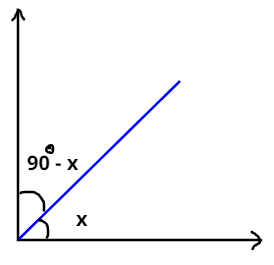Courses
Courses for Kids
Free study material
Free LIVE classes
MoreLIVE
Join Vedantu’s FREE Mastercalss

# Find the angle which is equal to its complement:Verified
362.7k+ views
Hint:- Sum of two complementary angles is ${90^0}$As we all know that if $\theta$ be an angle then,
Complement of $\theta$ will be $\left( {{{90}^0} - \theta } \right)$.
And the supplementary angle will be $\left( {{{180}^0} - \theta } \right)$.
So, we can say that the sum of two complementary angles is equal to ${90^0}$.
And the sum of two supplementary angles is equal to ${180^0}$.
Let the angle which had to find be x.
Then its complementary angle would be $\left( {{{90}^0} - x} \right)$.
As, given in the question that angle and its complementary angle will be equal. So,
$\Rightarrow x = \left( {{{90}^0} - x} \right)$ (1)
On, solving equation 1. We get,
$\Rightarrow 2x = {90^0}$
$\Rightarrow x = {45^0}$or $x = \dfrac{\pi }{4}$
Hence the angle which is equal to its complement will be ${45^0}$.
Note:- Whenever we came up with this type of problem then easiest and efficient way is to
go with the definition of complementary angles or supplementary angles, whichever is required. And after finding angles in degree we can also change that into radian.
Last updated date: 15th Sep 2023
Total views: 362.7k
Views today: 10.62k